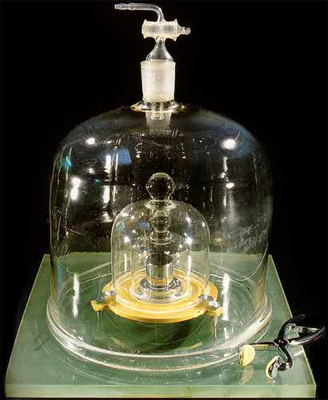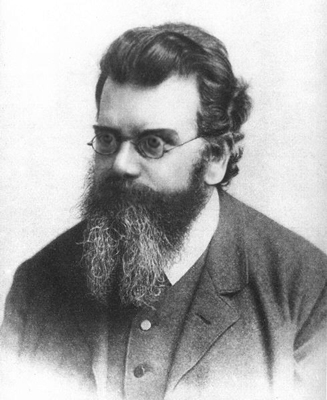﻿ Units: SI Fundamental Units

## Base Units of the International System (SI)

 The General Conference on Weights and Measures is nearing the end of a process to replace the definitions of its base (fundamental) units based on physical objects (such as standard meter sticks or standard kilogram bars) with definitions based physical constants which are thought to be immutable properties of the Universe. For those of us who are not physicists, these definitions seem abstract, but actually processes have been devised that allow them to be realized concretely in well-equiped laboratories. In recent years the kilogram has been the only base unit still defined by a physical object, a cylinder of 90% platinum and 10% iridium 39.17 mm (about 1.542 inches) in both height and diameter. It is seen inside the vacuum jars in the photo at right. The International Bureau of Weights and Measures (BIPM) keeps the world's International Prototype Kilogram in a vault in the basement of its building in Paris, and all other standards of mass, such as those of Britain and the United States, have been calibrated against this standard kilogram. Currently some 42 countries have approved copies of the prototype kilogram; there are three copies in the U.K. and five in the U.S. BIPM also keeps, for historical purposes, the International Prototype Meter, constructed at the same time as the prototype kilogram in 1889. However, it was retired as the official meter standard in 1960, as we'll see below. The physical standard for the kilogram resisted replacement because scientists can weigh objects very accurately. Mass standards in other countries can be adjusted to the Paris standard kilogram with an accuracy of one part per hundred million. The challenge was to figure out how to define the kilogram in any other way that can be reproduced with better accuracy than this. The 21st General Conference on Weights and Measures, meeting in October 1999, passed a resolution calling on national standards laboratories to press forward with research to "link the fundamental unit of mass to fundamental or atomic constants with a view to a future redefinition of the kilogram." It took at least 15 years to carry out this project. The 26th General Conference, meeting in November 2018, has adopted the revised standard for the kilogram as well as new definitions for the mole and the kelvin. Following are the official definitions of the seven base units, as given by BIPM.The 1889 International Prototype Kilogram BIPM photo

Time: The second (s) is the base unit of time. The second was originally defined as 1/86400 of a "mean solar day," as defined by astronomers. However, the rotation of the Earth is irregular and the length of the day is very slowly increasing, so this is not a satisfactory definition. In 1968 the 13th General Conference adopted this definition:

The second is the duration of 9 192 631 770 periods of the radiation corresponding to the transition between the two hyperfine levels of the ground state of the caesium 133 atom.

Length: The meter is the base unit of length. The meter was designed to represent one ten-millionth of the distance from the Equator to the North Pole. This distance was realized in practice as the length of a precious bar of platinum and iridium that is still kept by BIPM under carefully controlled conditions. In 1960 the 11th General Conference retired the International Prototype Meter bar and defined the meter to equal 1 650 763.73 wavelengths of the orange-red emission line in the electromagnetic spectrum of the krypton-86 atom in a vacuum. This definition held until 1983, when the 17th General Conference agreed to base the meter instead on the speed of light. The definition is:

The meter is the length of the path travelled by light in vacuum during a time interval of 1/299 792 458 of a second.

This means that the speed of light is declared officially to be exactly c = 299 792 458 meters per second. Clearly, this definition of the meter depends on the definition of the second.

Mass: The kilogram is the base unit of mass. The kilogram was designed to represent the mass of a liter of pure water at the point of freezing. This was realized in practice as the mass of a second precious bar of platinum and iridium, called the "international prototype kilogram" (IPK) that is still by BIPM under carefully controlled conditions. After years of research and discussion, this historic artifact has been retired. The 26th General Conference in November 2018 adopted this definition:

The kilogram is defined by taking the fixed numerical value of the Planck constant h to be 6.626 070 15 × 10−34 joule second.

The Planck constant is the "quantum of action," a fundamental value in quantum physics. Only Physics 101 is needed to show that the joule second is mathematically equivalent to the unit kg⋅m2⋅s, so knowing the Planck constant determines the kilogram in terms of the second and meter.

Electric current: The ampere is the base unit of electric current. The electrical units were initially defined the International Electrical Congress. The 9th General Conference in 1948 brought them into the International System, defining the ampere as "that constant current which, if maintained in two straight parallel conductors of infinite length, of negligible circular cross-section, and placed 1 meter apart in vacuum, would produce between these conductors a force equal to 2 × 10-7 newtons per meter of length." Since no one has conductors of infinite length, it was clear from the beginning that this definition could not be completely satisfactory. The 26th General Conference in November 2018 adopted this definition:

The ampere is defined by taking the fixed numerical value of the elementary charge e to be 1.602 176 634 × 10−19 coulomb.

This is a much simpler definition. The elementary charge is the charge on a single electron or proton. The coulomb is the SI unit of electric charge, and one ampere is a current of one coulomb per second. Thus it is really the coulomb that is defined, and its definition doesn't depend on any other unit definitions. The ampere is freed from dependence on the newton (the SI unit of force), but it still depends on the definition of the second.

 Thermodynamic temperature: The kelvin is the base unit for thermodynamic temperature (what is often called absolute temperature). The 10th General Conference in 1954 added this unit to the International System, defining it as "the fraction 1/273.16 of the thermodynamic temperature of the triple point of water." The triple point of water is the temperature and pressure (0.01 °C and 4.58 mm of mercury) at which water can exist simultaneously in the solid, liquid, and gaseous states. The 26th General Conference in November 2018 adopted this new definition: The kelvin is defined by taking the fixed numerical value of the Boltzmann constant k to be 1.380 649 × 10−23 joules per kelvin. The Boltzmann constant describes how the average kinetic energy of particles of a gas increases with increasing temperature, so it is naturally stated in energy units (joules) per kelvin. Joules per kelvin is mathematically equivalent to kg⋅m2⋅s−2⋅K−1, so the kelvin is determined from the Boltzmann constant once the kilogram, meter, and second are defined. Amount of substance: The mole is the base unit of amount of substance. The mole was added to the International System by the 14th General Conference in 1969. It was defined as "the amount of substance of a system which contains as many elementary entities as there are atoms in 0.012 kilogram (12 grams) of carbon 12." The "elementary entities" may be "atoms, molecules, ions, electrons, other particles, or specified groups of such particles." Of course, the number of atoms in 12 grams of carbon 12 is simply a whole number, known as Avogadro's number or the Avogadro constant. The 26th General Conference in November 2018 adopted this definition: The mole is defined by taking the fixed numerical value of the Avogadro constant NA to be exactly 6.022 140 76 × 1023. That's likely the largest precise number you've ever seen: There are now exactly 602 sextillion 214 quintillion 76 quadrillion atoms (or whatever) in a mole. On the nose. However, it's important to realize that we don't actually know how many atoms are in 12 grams of carbon 12. This new number is very close, relatively speaking, but the uncertainty is in the tens of quadrillions. Thus the new definition represents a relatively tiny, but unknown, change in the definition of the mole.Austrian physicist Ludwig Boltzmann (1844-1906) developed the kinetic theory of gases

Luminous intensity: The candela is the base unit of luminous intensity. This unit was first defined by the 9th General Conference in 1948, but the current definition was adopted by the 16th General Conference in 1979. It defines the candela as "the luminous intensity, in a given direction, of a source that emits monochromatic radiation of frequency 540 × 1012 hertz (540 terahertz) and that has a radiant intensity in that direction of 1/683 watt per steradian." The 26th General Conference in November 2018 adopted this definition, which is only a rewording of the present definition:

The candela is defined by taking the fixed numerical value of the luminous efficacy of monochromatic radiation of frequency 540 × 1012 hertz to be 683 lumens per watt.

Light of frequency 540 terahertz is green; this is approximately the frequency at which human eyes are most sensitive. The unit lumens per watt is mathematically equivalent to cd⋅sr⋅kg−1⋅m−2⋅s3, where cd is the symbol for the candela and sr is the symbol for the steradian, the mathematical unit of solid angle. Thus the candela is defined once the kilogram, meter, and second are defined.